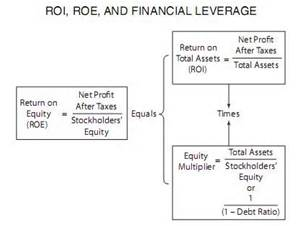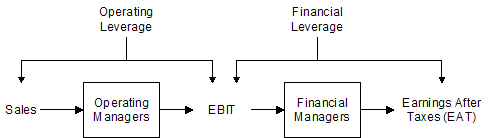Impact of Financial Leverage

jdhenning

This paper defines, describes, and illustrates financial leverage related to ROE and ROI using numerical illustrations.  A similar comparison of the effects and relationship of financial leverage is made with EBIT and Net Income using numerical illustrations.  Lastly a review of the article Lord (1998) is made evaluating the key elements of how time-series estimates impact the degree of leverage measures.

Financial Leverage Impact on ROE and ROI

Financial leverage relates to the level of debt or liabilities a company carries relative to the firm’s assets, income, investment, or revenue.  In one measurement called a debt ratio the proportion of total liabilities is divided by total assets to provide a view of the relative debt burden.  In another ratio of financial leverage called debt/equity ratio the proportion of total liabilities is divided by shareholders’ equity (Gibson, 2011).  These leverage ratios reveal a direct relationship to Return on Equity and Return on Investment by comparing components of the balance sheet (assets, liabilities, and equity) to the levels of income generated by a firm.  As the ROI-ROE chart illustrates, the Equity Multiplier or financial leverage represents the ratio of debt serving to increase ROA accordingly.Sander & Kantsukov (2009) most clearly explain the relationship by demonstrating that ROE will equal ROA when no financial leverage is present to act as an equity multiplier.  The following numerical examples relate these components of the balance sheet to assessing the leverage impact on ROE and ROI.  For example, a fictional company, Toys, Inc. may have \$30 million in net income and \$100 million in total shareholder equity that calculates to a very high 30% ROE.  This ROE is very positive in evaluating the performance of Toys, Inc., however it does not show the complete picture in relation to the company’s financial leverage.  Shareholder equity = Assets – Liabilities.  Leverage or debt creates an increase in both assets and liabilities, only by comparing the proportional growth of assets to liabilities can Toys, Inc. discover how well they are operating.  If Toys Inc. has \$5 billion in assets and \$4.9 billion in liabilities contributing to \$100 million in shareholder equity, the ROA will show a very different level of performance.  The ROA for Toys Inc. (\$30 million income / \$5 billion assets) is only 0.6% compared to 30% ROE.  While every shareholder dollar invested achieves a 30% return, every dollar invested in assets returned only 0.6% and may place Toys Inc. at dangerously high solvency risk with excessive financial leverage.  The debt to equity ratio in this example shows \$4.9 billion to \$100 million or a precariously high 49:1 debt burden.

Financial Leverage Impact on EBIT and Net Income

The impact of financial leverage on EBIT and Net Income is evidenced through a variety of measures beginning with decomposition factors and moving through to an analysis of degrees of leverage.  First, using ROE decomposition to compute the tax burden factor (Net income/EBT) is typically less than one in direct proportion to the tax rate of the company.  Likewise the interest burden factor (EBT/EBIT) is typically less than one in proportion to the cost of interest on liabilities.  These two factors of standard five factor decomposition illustrate how tax and interest burdens reduce or increase the firm’s profitability over time and are one way to identify trouble spots in the relationship of financial leverage to Net Income and EBIT.

Not only does financial leverage impact company health as illustrated through ROI and ROE decomposition analysis, but such leverage creates accelerating effects of burdens or benefits measured through percentage changes of decomposition ratios.  For example, the degree of financial leverage (DFL) can be measured by the percentage change in net income (or EAT in the chart) divided by the change in EBIT.  This measure of financial leverage is one way toview the accelerating effect or derivative of the percentage changes of the tax and interest factors place on the income (EAT) of the firm in the illustration above.

Another measure is the times interest earned ratio that provides a calculation of how many times over the company EBIT can pay the interest expense of financial leverage in a selected time period (Gibson, 2011).   In the Toys, Inc. example using a \$40 million EBIT and the lowest possible interest rates of 1% for expense on \$4.9 billion debt, the fictional interest expense of \$49 million overwhelms Toys, Inc. EBIT of \$40 million.  A times interest earned ratio of 40+49/49 or 1.8 times per year reflects the firm’s very weak long term ability to sustain all the financial leverage Toys, Inc. has incurred.

The fixed charge coverage is another financial leverage ratio that measures debt paying ability of a firm from the perspective of income statement components.  Like the times interest earned ratio the fixed charge coverage measures a broader scope of the firm’s fixed charges to its ability of earnings to cover those greater interests and charges to include the costs of operating leases (Gibson, 2011).  The important aspect of the coverage and times interest earned ratios is they provide estimated measures of the margin of liquidity on hand to cover financial leverage expenses as they come due in the time period analyzed.  It is also important to consider actual values in meaningful intervals to capture any periods where shortfalls may occur.  Calculations of average earnings or interest expenses over long time periods may serve to obscure periods of low income insufficient to meet interest expenses or operating lease costs as they come due (Gibson, 2011).

Review of Lord (1998) Article

Researcher Richard Lord seeks to explain why time series regression estimates of the degrees of operating leverage (DOL) and financial leverage (DFL) have a tendency to produce values less than one that run counter to theoretically expected outcomes.  Lord explains that firms operating above break-even levels should have DOL and DFL values greater than one because they are producing positive returns on effective leverage.  Such leverage is described by Garcia-Feijo and Jorgenson (2010) as DOL measures a firm’s reliance on fixed costs, while DFL measures a firm’s reliance on debt.

In support of Lord (1998), the Garcia-Feijo and Jorgenson (2010) study found that value firms tended to have much higher levels of DOL and DFL than growth firms and that the value firms covary more with recessions than growth firms.  Their research observed, “expected returns of value firms covary more with recessions than the returns of growth firms as value firms are less flexible than growth firms in adjusting to recessionary shocks” (p. 1129).

The counter-intuitive results that Lord (1998) investigates are strongest, “the higher above the break-even point a firm is operating” (p. 70).  Intuitively, the break-even point is higher for a firm the greater the reliance on fixed costs and debt.  So as Lord observed, external economic factors like recessionary shocks occur may pull the higher leveraged value firms down more significantly as evidenced in the unexpected time-series regression results of the study.  Lord (1998) also tests the point-to-point approach to estimate DOL and finds that just like time-series regression approach it suffers from a growth bias.

The study concludes that the time-series approach is gaining in prevalence and has less volatility than some alternative methodologies. However, the clear biases resulting from changes in operating values show a strong need to improve measures of operating and financial leverage and evaluate whether these measures actually gauge the degrees of leverage of a firm.

References
Garcia-Feijoo, L. & Jorgensen, R. D. (2010). Can operating leverage be the cause of the value premium?. Financial Management, Autumn (2010), 1127-1153.

Gibson, C. H. (2011).  Financial reporting & analysis: Using financial accounting information. Mason OH:   South-Western.   ISBN: 9781439080603

Lord, R. (1998). Properties of Time-Series Estimates of Degree of Leverage Measures.  Financial Review, 33, 69-84.

Sander, P. & Kantsukov, M. (2009). Effect of corporate taxation system on profitability and market ratios – the case of ROE and P/B ratios.  Research in Economics and Business: Central and Eastern Europe, 1(28), 27-40.